# Towards understanding IMU: Basics of Accelerometer and Gyroscope Sensors and How to Compute Pitch, Roll and Yaw Angles

An IMU chip contains accelerometer and gyroscope sensors, and it is referred to as 6-axis IMU or 6 DoF IMU. The IMU sometimes includes a magnetometer beside the accelerometer and gyroscope sensors, and in that case, it is referred to as 9-axis IMU or 9 DoF.

6-axis IMU is one of the most common sensors in electronic devices, and it is affordable and low-cost sensor. It gives important information about the device which allows us to build a wide range of applications. To understand the IMU we need to get a clear idea of the internal structure of the IMU and how it works. This understanding will help to define the advantages and disadvantages of each sensor inside the IMU. This will help to understand the signals we get from the IMU as well.

As I struggled personally to find a resource explaining practically everything needed in one place, I decided to start this series with the hope to start with showing a clear explanation of the IMU basics and ending the series with algorithms and applications important for IMU. Although Paul McWhorter’s 9-Axis Inertial Measurement Unit (IMU) series on Youtube is one of the best resources to understand IMU, but I failed to find written content with the same quality. That is why I’ve decided to build this article and extend on top of Paul’s work. Welcome to the first part of this topic series!

The applications of accelerometer and gyroscope sensors are varied, like: Gesture Recognition – Anomaly Detection for Predictive Maintenance – step counter – Indoor Positioning System. It is not strange to see such an important sensor in the Adidas smart ball for Qatar FIFA World Cup 2022.

We can not interpret the nature of the signals we read from the accelerometer and gyroscope output without understanding how both operate internally. We will not go deeply about the internal structure, but we will explain what is needed to reach the anticipated understanding. IMU which stands for inertial measurement unit is built with MEMS technology which stands for Microelectromechanical systems.

## How does Accelerometer work?

Accelerometer is based on a moving mass fixed to a frame with a spring (in reality it is not a spring). This mass can move along one axis only, and the accelerometer has 3-axis.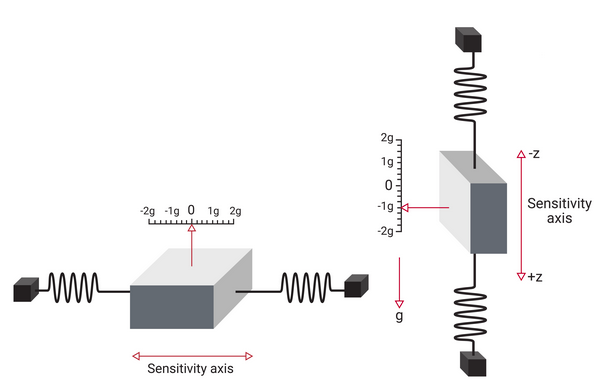An illustration of the basic internal structure inside the accelerometer which is a mass with springs attached to a frame. Image source: Vectornav

The mass is responsible for changing the value of a variable capacitor formulated between a fixed electrode and the surface of the moving mass. We know from electricity basics that the capacity value in Farad is expressed using electrode surface area A and distance D between electrodes.

C = (ε × A)/D (Farad)

The external force (caused by acceleration) applied to the mass will change the distance D. By measuring the resultant capacitor, we can find D change which is proportional to acceleration. According to second Newton’s law, the force vector is equal to the mass multiplied by the acceleration. This explaines how the variable capacity can be mapped with the physical acceleration. Practically, the datasheet provides a number maps the digital reading with the accelerometer value and this is known as sensitivity.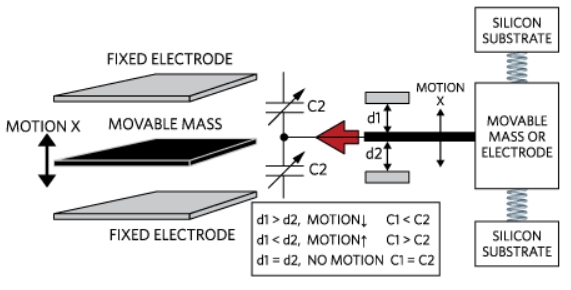The moving mass causes a change in the formed capacitor between the fixed electrode and the moving surface of the mass. The image source: APPLICATION NOTE 5830 from Maxim Integrated

The capacity of the variable capacitor is measured using an ADC. To increase the capacity and sensitivity, the moving mass and fixed frame are designed to have more than one variable capacitor attached in parallel inside the internal design of the accelerometer.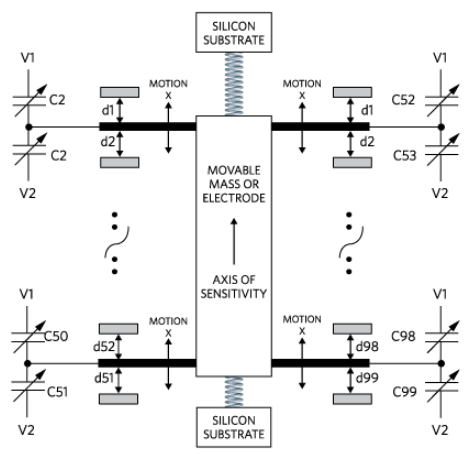More than one variable capacitor formed with the moving mass are attached on parallel to get higher capacity and more sensitivity. Image source: APPLICATION NOTE 5830 from Maxim Integrated

But how is the physical acceleration value mapped to the measured capacity value? The ADC returns a numeric value that expresses the voltage across the capacitor. By using the reference value of the voltage in the steady state (no motion), we can find the voltage difference. By using the sensitivity value which maps the change of voltage for each 1g change to voltage change, we can find acceleration value.

An example adapted from APPLICATION NOTE 5830 from Maxim Integrated

Let’s say the IMU 10-bit ADC powered from 3.3V returned a digital value of 600, then the voltage value is:

600 x 3.3 / 1023 = 1.94V

We know that the no motion state equals 1.65V, then the difference is:

1.94V – 1.65V = 0.29V

The sensitivity from the datasheet is 0.475V/g which means that each 0.475V change equals 1g. We find:

0.29V/0.475V/g = 0.6g

Practically, in digital IMU, the sensitivity value is matched per LSB as shown below for an example datasheet of BMX160.

The image below shows an illustration of what the internal structure of accelerometer looks like.

### Why does an accelerometer give a non-zero value with no motion?

The accelerometer shows a non-zero value over the z-axis when it is on a flat plane with no motion state. This is because of the earth’s gravity g which equals 9.8 m/s2 . When the accelerometer is in a free fall state, the value over the z-axis will be zero as the mass will be under a force equal to the earth’s gravity in the opposite direction. The understanding of this behaviour will not be possible without understanding the accelerometer structure shown previously.

The accelerometer senses the acceleration caused by the earth’s gravity and any other external forces. The read from the accelerometer output is expressed below with added measurement noise with a Gaussian distribution.$\widetilde{a}=a^{(g)}+a^{(l)}+\eta_{a c c}, \quad \eta_{a c c} \sim \mathcal{N}\left(0, \sigma_{a c c}^2\right)$

## How Does Gyroscope Work?

What has been shown so far was for the accelerometer sensor. Gyroscope shares a similar way of having a moving mass inside but in a different mechanical design. The main difference is that Gyroscope relies on Coriolis Effect, so it utilizes a resonating moving mass and whenever there is an external effector. The angular velocity is calculated using the Coriolis Effect.

The need for a constantly resonating moving mass makes the power consumption of the Gyroscope higher than the accelerometer. This can be noted from the supply currents table from the IMU datasheet.A comparison of three different IMUs each from different manufacturer. It is clearly showing that Gyroscope draws more current than the accelerometer.

The Gyroscope senses the angular velocity in deg/sec with a drift added beside the measurement noise. We will find later more about the drift issue.$\widetilde{\omega}=\omega+b+\eta_{\text {gyro }}, \quad \eta_{\text {gyro }} \sim \mathcal{N}\left(0, \sigma_{\text {gyro }}^2\right)$

## Computing the Pitch and Roll Angles Using Accelerometer

Pitch and roll angles are one of the most important pieces of information extracted from the IMU sensor output. Pitch angle is formed between the nose of the body and the flat surface. It is similar to the position of an airplane taking off and landing. Roll angle is formed between the body and the flat surface by rolling the body left and right. It is similar to the position of the airplane rolling while flying.

The gravity vector is perpendicular to x-axis and y-axis which makes the value on x and y equal to zero. Gravity when the sensor body is on a flat surface will affect the z-axis only. When we pitch the body with angle Θ, the value on x-axis is no more zero as the g vector is no more perpendicular to it, while the g vector is still perpendicular to y-axis.

From geometry rules, we find that the Pitch angle Θ between the body and the surface is equal to the angle between g vector and the z-axis after pitching. The Θ angle is complementary to the angle formed between the g vector and the x-axis.

By using the z-axis and x-axis values we find Θ :$tan(\theta)=\frac{a_x}{a_z} \Rightarrow \theta = tan^{-1}(\frac{a_x}{a_z} )$

Similarly, we find the rolling angle using the z-axis and y-axis:$tan(\phi)=\frac{a_y}{a_z} \Rightarrow \phi = tan^{-1}(\frac{a_y}{a_z} )$An Illustration for the Θ angle showing how it is complementary to the angle formed between the g vector and the x-axis.

In the following code using break-out board GY-512 of MPU-6050 IMU, we calculate the pith and roll angles using the previous equations:

You will find in the following code conversion from rad to deg as atan2 returns the value in rad. Also, remember that 1 g equals to 9.8 m/s2
#include <Adafruit_MPU6050.h>
#include <Wire.h>
#include <math.h>

void setup(void) {
Serial.begin(115200);
while (!Serial)
delay(10); // will pause Zero, Leonardo, etc until serial console opens

// Try to initialize!
if (!mpu.begin()) {
Serial.println("Failed to find MPU6050 chip");
while (1) {
delay(10);
}
}
Serial.println("MPU6050 Found!");

mpu.setAccelerometerRange(MPU6050_RANGE_8_G);
Serial.print("Accelerometer range set to: ");
switch (mpu.getAccelerometerRange()) {
case MPU6050_RANGE_2_G:
Serial.println("+-2G");
break;
case MPU6050_RANGE_4_G:
Serial.println("+-4G");
break;
case MPU6050_RANGE_8_G:
Serial.println("+-8G");
break;
case MPU6050_RANGE_16_G:
Serial.println("+-16G");
break;
}
mpu.setGyroRange(MPU6050_RANGE_500_DEG);
Serial.print("Gyro range set to: ");
switch (mpu.getGyroRange()) {
case MPU6050_RANGE_250_DEG:
Serial.println("+- 250 deg/s");
break;
case MPU6050_RANGE_500_DEG:
Serial.println("+- 500 deg/s");
break;
case MPU6050_RANGE_1000_DEG:
Serial.println("+- 1000 deg/s");
break;
case MPU6050_RANGE_2000_DEG:
Serial.println("+- 2000 deg/s");
break;
}

Serial.println("");
delay(100);
}

float theta;
float phi;

void loop() {

/* Get new sensor events with the readings */
sensors_event_t a, g, temp;
mpu.getEvent(&a, &g, &temp);

theta=atan2(a.acceleration.x/9.8,a.acceleration.z/9.8)/2/3.141592654*360;
phi=atan2(a.acceleration.y/9.8,a.acceleration.z/9.8)/2/3.141592654*360;

Serial.print(":theta:");
Serial.print(theta);
Serial.print(",");
Serial.print("phi:");
Serial.println(phi);

delay(50);
}


### Limitation of calculating the angles using an accelerometer and improving it using a filter

Calculating pitch and roll angles using accelerometer suffers from vibrations even without changing the angle itself. Having vibrations is the normal case of utilizing the IMU in the real-world. This makes the previous equations suitable for simple cases and simple applications.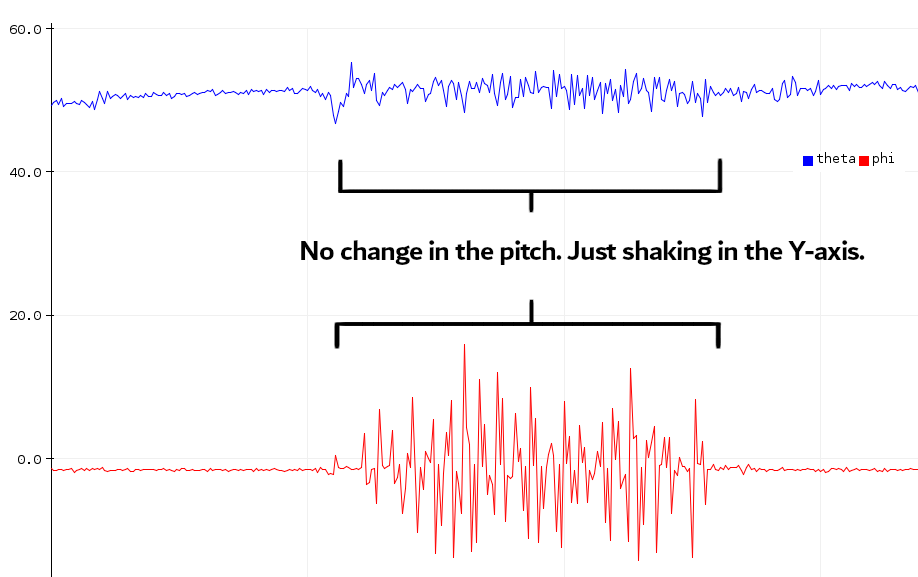In this example, we have a 45 degree pitch and by shaking the board in the Y-axis direction only we find that the pitch was sensitive to the vibration on the y-axis although the x-axis and z-axis should not affect in theory.

We can enhance the quality of the calculated pitch signal by adding a 1’st degree low-pass filter:$\theta_{old} = 0.9 * \theta_{old} + 0.1 * \theta_{new}$

We modify the previous code and add the low-pass filter part as follows:

theta_new = 0.9*theta_old + 0.1* theta;
phi_new = 0.9*phi_old + 0.1* phi;
//Serial.print(a.timestamp);

Serial.print("theta_raw:");
Serial.print(theta);
Serial.print(",");
Serial.print("phi_raw:");
Serial.print(phi);
Serial.print(",");
Serial.print("theta_filter:");
Serial.print(theta_new);
Serial.print(",");
Serial.print("phi_filter:");
Serial.println(phi_new);

theta_old = theta_new;
phi_old = phi_new;
delay(50);


The scalar values 0.9 and 0.1 are constants that need to be fine-tuned depending on the speed of response and vibration rejection needed from the output. The summation of both should be equal to 1. For example, 0.9 means that we trust the old computed value more than the new one. This will make the response slower but with more immunity to vibration.

## Computing the pitch and roll angle using Gyroscope

As the Gyroscope provides the angular rate deg/sec, we can extract back the angle information from the Gyroscope by multiplying the output with the time difference of the sequence of reading. We consider the initial angle as zero.$\theta = \theta_{old} + \omega_y * dt$$\phi = \phi_{old} + \omega_x * dt$

We can calculate the time difference between each reading using a timer in case our Gyroscope does not provide the timestamp information with the reading which is a rare case for new IMU designs.

We can notice that the pitch angle computed using Gyroscope is less sensitive to vibration compared to the accelerometer case.The computed pitch angle using accelerometer (blue) looks more sensitive to vibration on the y-axis than computed pitch angle using Gyroscope (red). The Gyroscope suffers from drift issue while the accelerometer does not.
// Acc
float theta_acc;
float phi_acc;

//Gyro
float theta_gyro;
float phi_gyro;

float theta_gyro_old = 0.0;
float phi_gyro_old = 0.0;

float theta_gyro_new;
float phi_gyro_new;

int32_t last_timestamp = 0;
float delta_time = 0;

void loop() {

/* Get new sensor events with the readings */
sensors_event_t a, g, temp;
mpu.getEvent(&a, &g, &temp);

theta_acc=atan2(a.acceleration.x/9.8,a.acceleration.z/9.8)/2/3.141592654*360;
phi_acc=atan2(a.acceleration.y/9.8,a.acceleration.z/9.8)/2/3.141592654*360;

delta_time =  (millis()-last_timestamp)/1000.;
last_timestamp  = millis() ;

theta_gyro = theta_gyro + g.gyro.y * delta_time * 360 / (2*3.141592654) ; // rad/sec
phi_gyro = phi_gyro + g.gyro.x * delta_time * 360 / (2*3.141592654) ; // rad/sec

Serial.print("theta_acc:");
Serial.print(theta_acc);
Serial.print(",");
Serial.print("theta_gyro:");
Serial.println(-1* theta_gyro );

theta_acc_old = theta_acc_new;
phi_acc_old = phi_acc_new;
delay(100);
}

The provided codes are not optimal, where it is more accurate to use the data ready interrupt to find the reading time or to use the timestamp information from each reading, if supported in the IMU registers. However, for MPU6050, the timestamp is not available. We will use the millis() timer for now.

The reason behind the robustness of computed angles through Gyroscope against vibrations is  because of the integration we are doing by multiplying the angular rate with time.$\int \cos (2 \pi f t)=\frac{1}{2 \pi f} \sin (2 \pi f t)$

This means that the noise (vibration) signal is suppressed by 1\2*pi*f and by increasing the Gyroscope frequency we increase the signal quality.

### Limitation of computing the angles using a Gyroscope

Using the Gyroscope to compute the angles is not an optimal solution. We need to calibrate it before using it. The calibration will make the drift minimal. What we have seen in the last figure is that due to the drift value, the angle keeps accumulating over time although the board was steady on the table. The calibration will reduce this drifting as much as possible.

Computing the angle using the discussed way with Gyroscope has an approximation error due to using first-order Taylor series  :$\theta(t+\Delta t) \approx \theta(t)+\frac{\partial}{\partial t} \theta(t) \Delta t+\epsilon$

This error can be reduced by increasing the Gyroscope frequency.

Remember that:$\mathrm{rad} / \mathrm{s}=\frac{360}{2 \pi} \mathrm{deg} / \mathrm{s}=57.2957795 ~\mathrm{deg} / \mathrm{s}$ and$\text { degress }/\mathrm{s}=\frac{2 \pi}{360} \mathrm{rad} / \mathrm{s}=0.01745329251~ \mathrm{rad} / \mathrm{s}$

### Gyroscope calibration

We found previously that drift affects the computed angles from Gyroscope readings. Calibration will make sure to reduce the drift value to the minimum. One of the straightforward ways of calibrating the Gyroscope is to make the board in no motion state and then take readings and extract the maximum and minimum and then find the average. We deduct the calculated average from each reading. Adafruit offers a Python script to calibrate Gyroscope and Magnetometer. To make things easier, the script is modified to calibrate Gyroscope only.

The script listens to the port and expects to receive in each line 3 values of x,y, and z separated by a comma and ended with \r\n. The script will generate 3 values as a result.

# A modified version of: https://learn.adafruit.com/adafruit-sensorlab-gyroscope-calibration?view=all
import time
%matplotlib notebook
import matplotlib.pyplot as plt
from matplotlib.animation import FuncAnimation
import datetime
import matplotlib.dates as mdates
from collections import deque
import numpy as np

import serial
import re

PORT = "/dev/ttyACM0"

# How many sensor samples we want to store
HISTORY_SIZE = 2500

# Pause re-sampling the sensor and drawing for INTERVAL seconds
INTERVAL = 0.01

serialport = None

def get_imu_data():
global serialport
if not serialport:
# open serial port
serialport = serial.Serial(PORT, 115200, timeout=0.1)
# check which port was really used
print("Opened", serialport.name)
# Flush input
time.sleep(3)

# Poll the serial port
if not line:
return None
vals = line.strip().split(',')
#print(vals)
if len(vals) != 3:
return None
try:
vals = [float(i) for i in vals]
except ValueError:
return None
#print(vals)
return vals

print("Put down the board and do not touch or move it!")
for s in range(3, 0, -1):
print(s, end='...')
time.sleep(1)
print("COLLECTING GYRO DATA")

# close port in case its open
if serialport:
try:
serialport.close()
except NameError:
pass

serialport = None

# Deque for axes
gyro_x = deque(maxlen=HISTORY_SIZE//10)
gyro_y = deque(maxlen=HISTORY_SIZE//10)
gyro_z = deque(maxlen=HISTORY_SIZE//10)
while len(gyro_x) < (HISTORY_SIZE//10):
ret = get_imu_data()
#print(ret)
if not ret:
continue
x, y, z = ret[0:3]
gyro_x.append(x)
gyro_y.append(y)
gyro_z.append(z)

for _ in range(3):
gyro_x.popleft()
gyro_y.popleft()
gyro_z.popleft()

min_x = min(gyro_x)
max_x = max(gyro_x)
min_y = min(gyro_y)
max_y = max(gyro_y)
min_z = min(gyro_z)
max_z = max(gyro_z)

print("Gyro X range: ", min_x, max_x)
print("Gyro Y range: ", min_y, max_y)
print("Gyro Z range: ", min_z, max_z)

gyro_calibration = [ (max_x + min_x) / 2, (max_y + min_y) / 2, (max_z + min_z) / 2]
print("Final calibration in deg/s:", gyro_calibration)

serialport.close()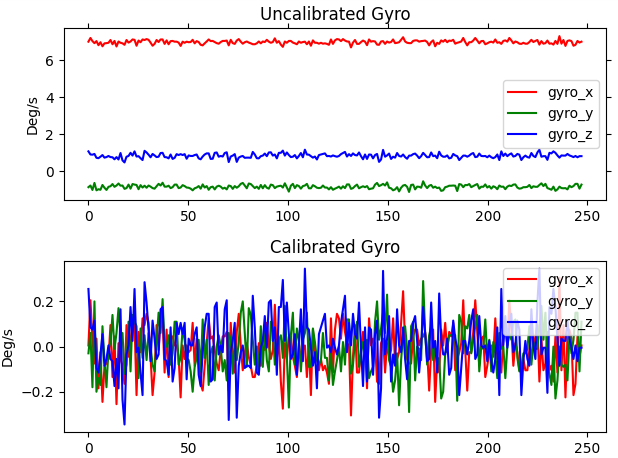An example of the MPU6050’s Gyroscope output before and after the calibration. We can see how the signals across the 3 axes are centered around zero.

We notice in the figure below how the computed pitch angle is not drifting like pre-calibration.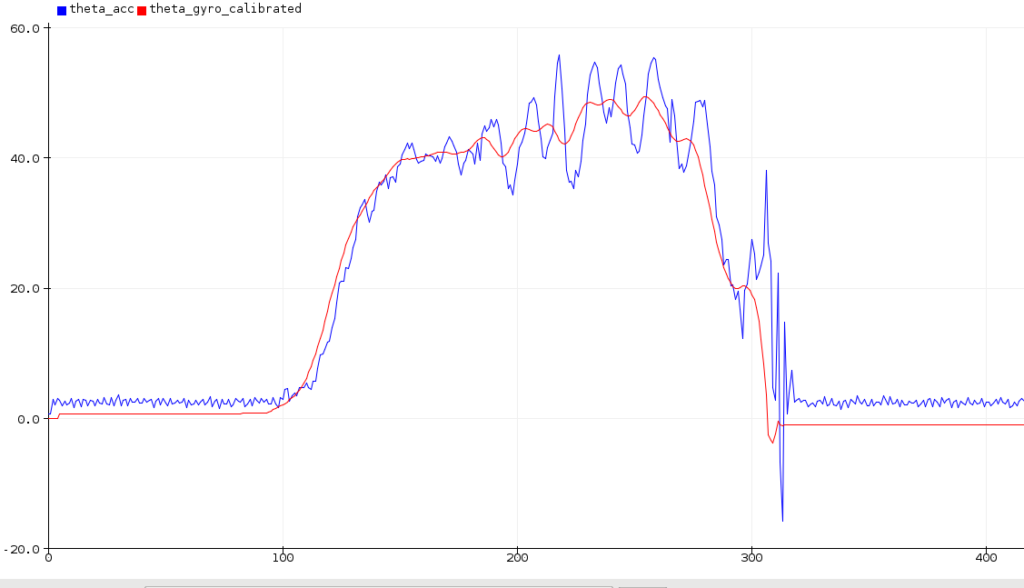An example signal of the pitch angle computed from the accelerometer (blue) and computed using the calibrated Gyroscope (red). We can see that the red line is not drifting, like before, after doing the calibration.

We modify the Arduino code to utilize the generated value of the calibration script

cal_gyro_x = g.gyro.x* 360 / (2*3.141592654) - 7.005 ;
cal_gyro_y = g.gyro.y* 360 / (2*3.141592654) + 0.84 ;
cal_gyro_z = g.gyro.z* 360 / (2*3.141592654) - 0.815 ;

theta_gyro = theta_gyro + cal_gyro_y * delta_time  ; // deg/sec
phi_gyro = phi_gyro + cal_gyro_x * delta_time  ; // deg/sec


## Which is better? accelerometer or Gyroscope?

As we saw in previous sections, the accelerometer is affected less by drift problems, but it is more sensitive to vibrations. Gyroscope is more resistant to vibrations but way more affected by drift issue. To get benefits of both worlds, we may use a complementary filter.$\theta^{(t)}=\alpha\left(\theta^{(t-1)}+\widetilde{\omega} \Delta t\right)+(1-\alpha) {atan} 2\left(\widetilde{a}_x, \widetilde{a}_z\right)$

We fine-tune the filter parameters to give weight to what more important to us; the response time or immunity to vibrations.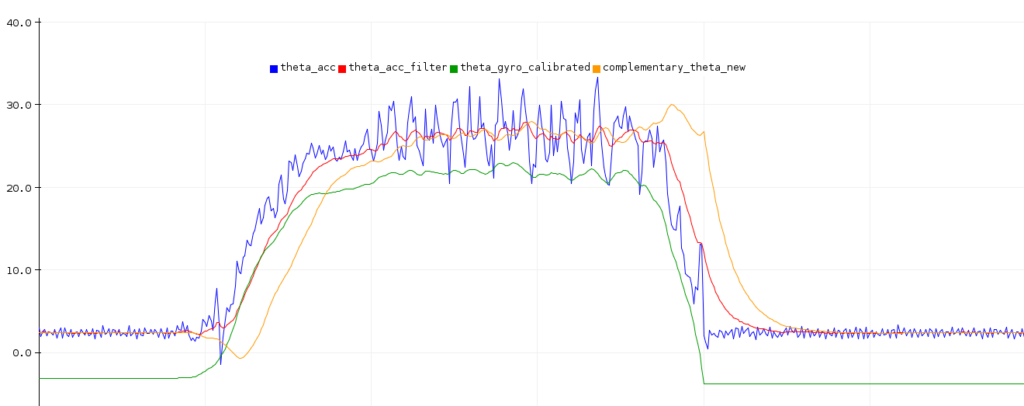A comparison of the different ways to calculate the pitch angle using: Accelerometer without filter (blue), accelerometer with a low-pass filter (red), calibrated Gyroscope (green) and complementary filter (yellow)

You can use the code below to compare the different methods

// Acc
float theta_acc;
float phi_acc;

float theta_acc_old = 0.0;
float phi_acc_old = 0.0;

float theta_acc_new;
float phi_acc_new;

//Gyro
float theta_gyro;
float phi_gyro;

float theta_gyro_old = 0.0;
float phi_gyro_old = 0.0;

float theta_gyro_new;
float phi_gyro_new;

float cal_gyro_x;
float cal_gyro_y;
float cal_gyro_z;

int32_t last_timestamp = 0;
float delta_time = 0;

float complementary_theta_old = 0.0 ;
float complementary_theta_new ;

float complementary_phi_old = 0.0 ;
float complementary_phi_new ;

void loop() {

/* Get new sensor events with the readings */
sensors_event_t a, g, temp;
mpu.getEvent(&a, &g, &temp);

//  /* Print out the values */
//  Serial.print("Acceleration X: ");
//  Serial.print(a.acceleration.x);
//  Serial.print(", Y: ");
//  Serial.print(a.acceleration.y);
//  Serial.print(", Z: ");
//  Serial.print(a.acceleration.z);
//  Serial.println(" m/s^2");
//
//  Serial.print("gyro_x:");
//  Serial.print(g.gyro.x* 360 / (2*3.141592654));
//  Serial.print(",");
//  Serial.print("gyro_y:");
//  Serial.print(g.gyro.y* 360 / (2*3.141592654));
//  Serial.print(",");
//  Serial.print("gyro_z:");
//  Serial.print(g.gyro.z* 360 / (2*3.141592654));
//  Serial.print(",");
// calibration [7.005, -0.84, 0.815]

cal_gyro_x = g.gyro.x* 360 / (2*3.141592654)- 7.005 ;
cal_gyro_y = g.gyro.y* 360 / (2*3.141592654) + 0.84 ;
cal_gyro_z = g.gyro.z* 360 / (2*3.141592654) - 0.815 ;

//  Serial.print("cal_gyro_x:");
//  Serial.print(cal_gyro_x);
//  Serial.print(",");
//  Serial.print("cal_gyro_y:");
//  Serial.print(cal_gyro_y);
//  Serial.print(",");
//  Serial.print("cal_gyro_z:");
//  Serial.println(cal_gyro_z);

//Serial.print("Ax:");
//Serial.print(a.acceleration.x);
//Serial.print(",");
//Serial.print("Ay:");
//Serial.print(a.acceleration.y);
//Serial.print(",");
//Serial.print("Az:");
//Serial.println(a.acceleration.z);

theta_acc=atan2(a.acceleration.x/9.8,a.acceleration.z/9.8)/2/3.141592654*360;
phi_acc=atan2(a.acceleration.y/9.8,a.acceleration.z/9.8)/2/3.141592654*360;

theta_acc_new = 0.9*theta_acc_old + 0.1* theta_acc;
phi_acc_new = 0.9*phi_acc_old + 0.1* phi_acc;
//Serial.print(a.timestamp);

delta_time =  (millis()-last_timestamp)/1000.;
last_timestamp  = millis() ;

//Serial.print("delta_time:");
//Serial.print(delta_time);
//Serial.print(",");

theta_gyro = theta_gyro + cal_gyro_y * delta_time  ; // deg/sec
phi_gyro = phi_gyro + cal_gyro_x * delta_time  ; // deg/sec

complementary_theta_new = 0.5 * (complementary_theta_old + cal_gyro_y * delta_time) + 0.5 * theta_acc_new ;
complementary_phi_new = 0.5 * (complementary_phi_old + cal_gyro_x * delta_time) + 0.5 * phi_acc_new ;

Serial.print("theta_acc:");
Serial.print(theta_acc);
Serial.print(",");
//Serial.print("phi_raw:");
//Serial.print(phi_acc);
//Serial.print(",");
Serial.print("theta_acc_filter:");
Serial.print(theta_acc_new);
Serial.print(",");
//Serial.print("phi_acc_filter:");
//Serial.println(phi_acc_new);

Serial.print("theta_gyro_calibrated:");
Serial.print(-1* theta_gyro );
Serial.print(",");
//Serial.print("phi_gyro:");
//Serial.println(phi_gyro);

Serial.print("complementary_theta_new:");
Serial.println(complementary_theta_new );

theta_acc_old = theta_acc_new;
phi_acc_old = phi_acc_new;

complementary_theta_old = complementary_theta_new;
complementary_phi_old = complementary_phi_new;
delay(10);
}


## Computing yaw angle

We have discussed so far computing pitch and roll angles. Yaw angle can be computed using compass and Gyroscope only. The accelerometer can be used to compute pitch and roll angles only as the gravity vector is perpendicular to x-axis and y-axis while parallel to z-axis. Yawing the body over the z-axis will not make any change on any axis of the accelerometer. The compass can find the yaw angle using the earth’s magnetic field. We have discussed how to calibrate and find the geographic direction using a compass sensor in a past article

We will use the Gyroscope to calculate the yaw which is the value change over z-axis.

We modify the code to extract the yaw angle using the calibrated Gyroscope:

cal_gyro_x = g.gyro.x* 360 / (2*3.141592654) - 7.005 ;
cal_gyro_y = g.gyro.y* 360 / (2*3.141592654) + 0.84 ;
cal_gyro_z = g.gyro.z* 360 / (2*3.141592654) - 0.815 ;

theta_gyro = theta_gyro + cal_gyro_y * delta_time  ; // deg/sec
phi_gyro = phi_gyro + cal_gyro_x * delta_time  ; // deg/sec
yaw_gyro = yaw_gyro + cal_gyro_z * delta_time  ; // deg/sec


### Yahya Tawil

Embedded Hardware Engineer interested in open hardware and was born in the same year as Linux. Yahya is the editor-in-chief of Atadiat and believes in the importance of sharing free, practical, spam-free and high quality written content with others. His experience with Embedded Systems includes developing firmware with bare-metal C and Arduino, designing PCB&schematic and content creation.

This site uses Akismet to reduce spam. Learn how your comment data is processed.